Open In App

# How to Calculate Mean Absolute Percentage Error in Excel?

In statistics, we often use Forecasting Accuracy which denotes the closeness of a quantity to the actual value of that particular quantity. The actual value is also known as the true value. It basically denotes the degree of closeness or a verification process that is highly used by business professionals to keep track records of their sales and exchanges to maintain the demand and supply mapping every year. There are various methods to calculate Forecasting Accuracy.

So, one of the most common methods used to calculate the Forecasting Accuracy is MAPE which is abbreviated as Mean Absolute Percentage Error. It is an effective and more convenient method because it becomes easier to interpret the accuracy just by seeing the MAPE value.

In this article, we are going to see how to calculate Mean Absolute Percentage Error, MAPE in Excel.

The formula to calculate MAPE is :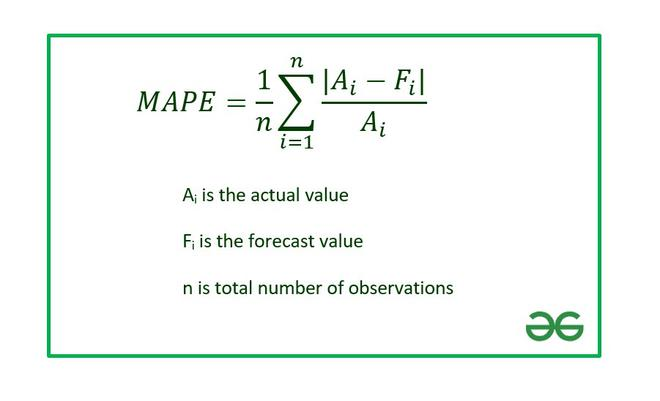The above formula can be interpreted as the average value of Absolute Percentage Error (APE) of all the observations in the data set.

Note: The actual value can’t be zero. We can observe from the above formula that if the Actual value becomes zero, it will be undefined.

Example:

Consider the dataset shown below :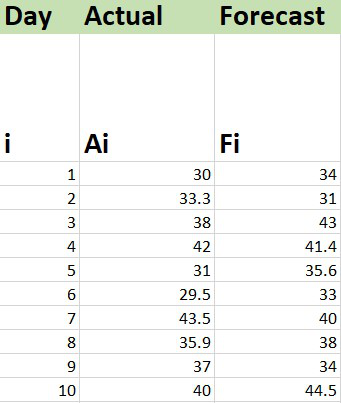### Calculation of MAPE in Excel:

The functions needed for formulas in Excel are-

ABS : To calculate the absolute value.

AVERAGE : To calculate the mean.

The steps are :

1. Insert the data set in the Excel sheet.

2. Calculate APE for each individual observation using Excel Formula. The formula will be :

```=ABS(Cell_No_Act-Cell_No_Fore)/Cell_No_Act*100

where
ABS : Used to calculate the absolute value
Cell_No_Act : Cell number where Actual value is present
Cell_No_Fore : Cell number where Forecast value is present```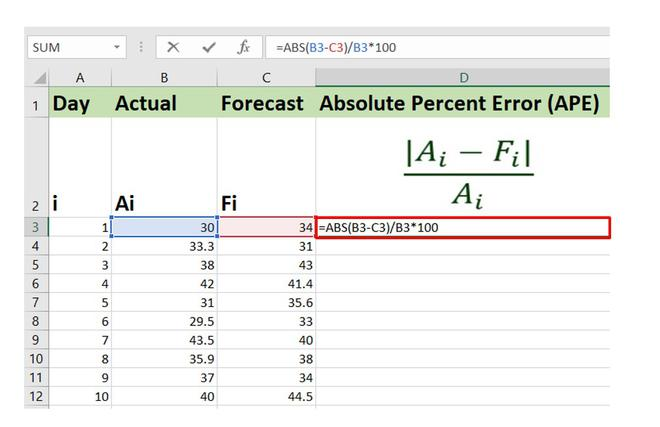Similarly, you can write the formulas for the other entries and get the APE for all the records.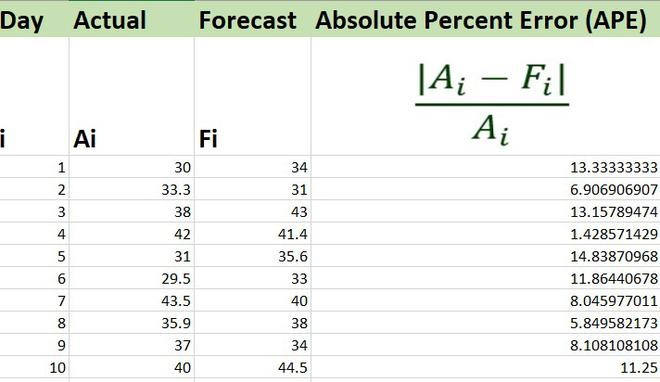APE

3. Now, simply we need to find the average or the mean value for all these values in order to calculate MAPE.

The formula to find average value in Excel is :

`=AVERAGE(Cell_Range)`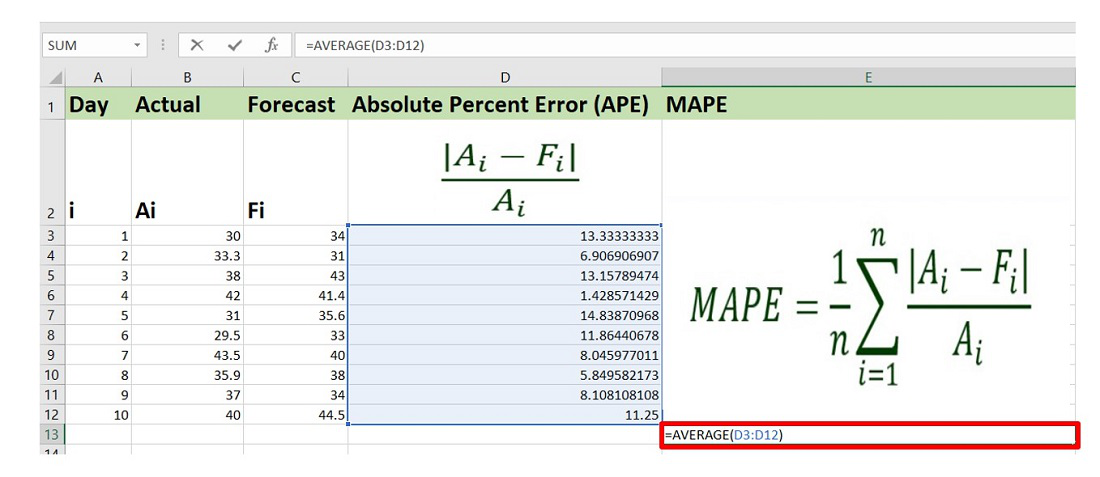The value of MAPE for the given data set is 9.478% approximately. Therefore, we can say that  the average difference between the actual value and forecasted value is 9.478%.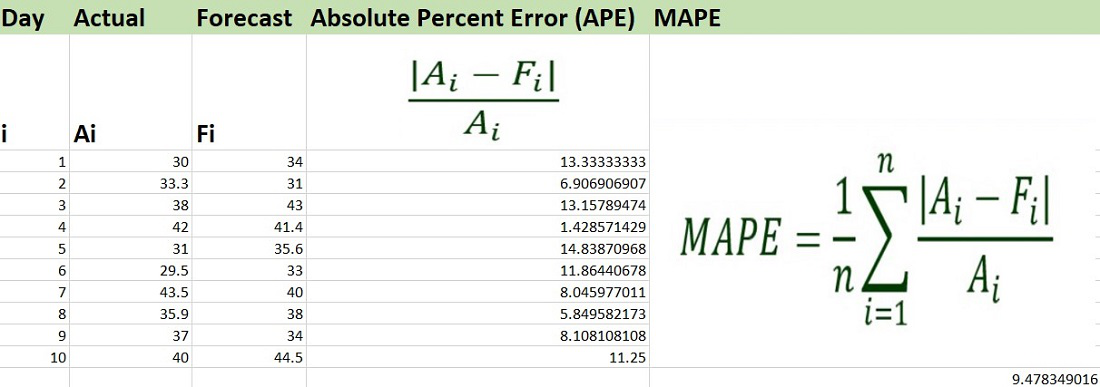MAPE